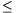Mathematical and Physical Journal
for High Schools
Issued by the MATFUND Foundation
 Already signed up? New to KöMaL?# Exercises and problems in InformaticsSeptember 2001

Sz. 1. We learned from an article of József Bölcsföldi and Géza Balázs (KöMaL Homepage Dec. 2000) that already the Pythagoreans knew about numbers that form a friendly pair--which is a pair (a,b) of natural numbers with both numbers being equal to the sum of the proper divisors (i.e. including 1 but excluding the number itself) of the other. Clearly, one number in a friendly pair has many divisors (typed in bold below), while the other one has only few of them. Some friendly pairs include (220, 284),  (1184, 1210),  (2620, 2924),  (5020, 5564),  (6232, 6368),  (10744, 10856), ...'' Write a program which asks for two natural numbers (N<M<106), then prints all the friendly pairs (a,b) with N<a, b<M.  (10 points)

Sz. 2. In most programming languages an ellipse can be displayed by a single command, however this ellipse must have axes parallel to the edges of the screen. Write a program that enables us to draw the ellipse in any position. The parameters are the length of the axes and the angle between the major axis and the upper edge of the screen in degrees.  (10 points)

Sz. 3. We deposit our savings for N years (1N$\displaystyle le$10) according to the following: 1. Throughout N years, at the beginning of every month we place A Forints into the bank, and lock it up for one year at a rate of interest X percent (X>0 a real number). 2. When the lockup is over, at the beginning of the next month we get the yearly interest, which is put back and locked up again for one year together with our savings so far. Make a sheet (named PENZ.XLS) which the numbers N, A and X can be entered into, then our bank balance is calculated throughout N years. The sheet should contain exactly N rows, that is unnecessary rows should not be visible even if N is changed. Example (with N=3, A=1000, X=10)

 1st year: 1000 2000 3000 4000 5000 6000 7000 8000 9000 10000 11000 12000 2nd year: 13100 14200 15300 16400 17500 18600 19700 20800 21900 23000 24100 25200 3rd year: 26410 27620 28830 30040 31250 32460 33670 34880 36090 37300 38510 39720

(10 points)